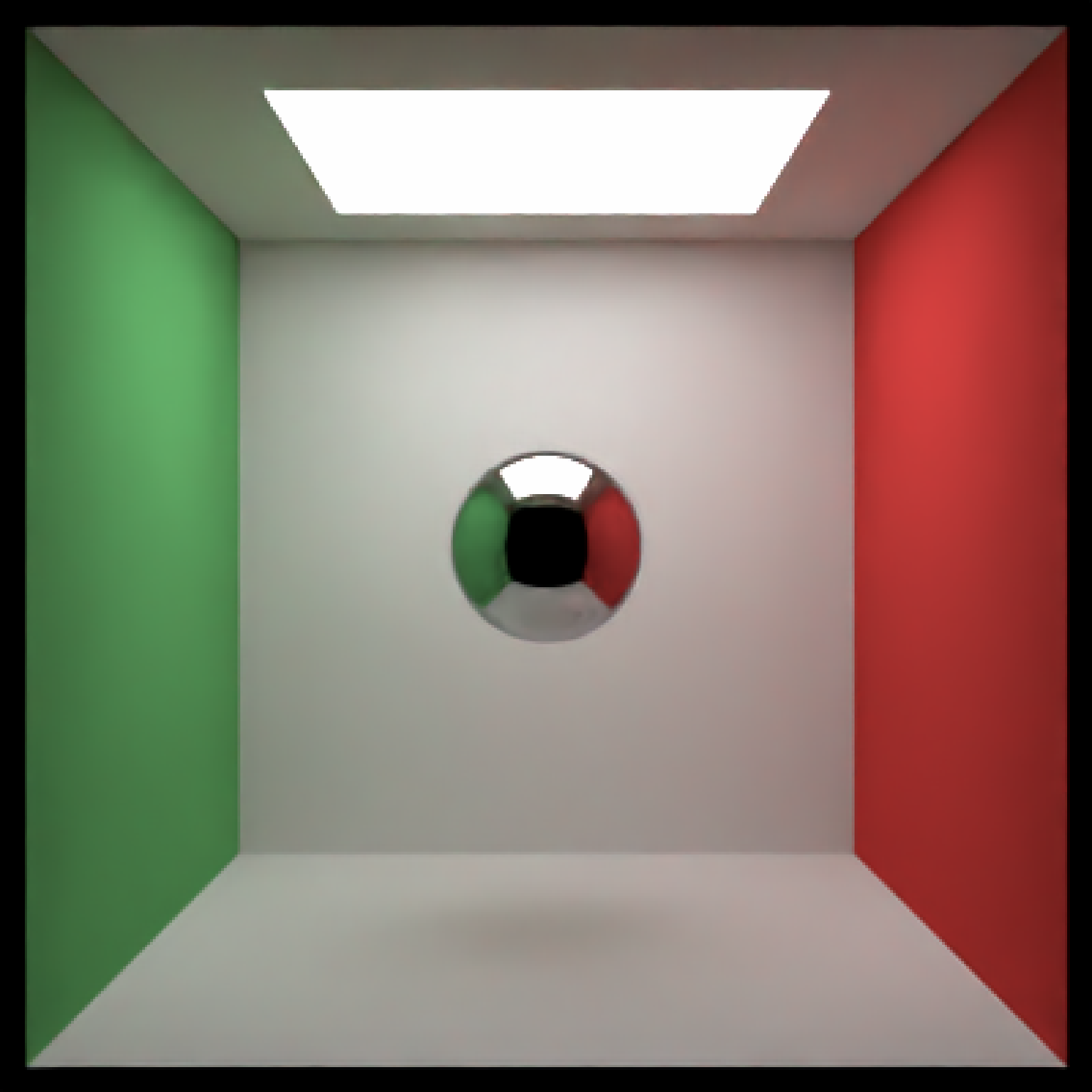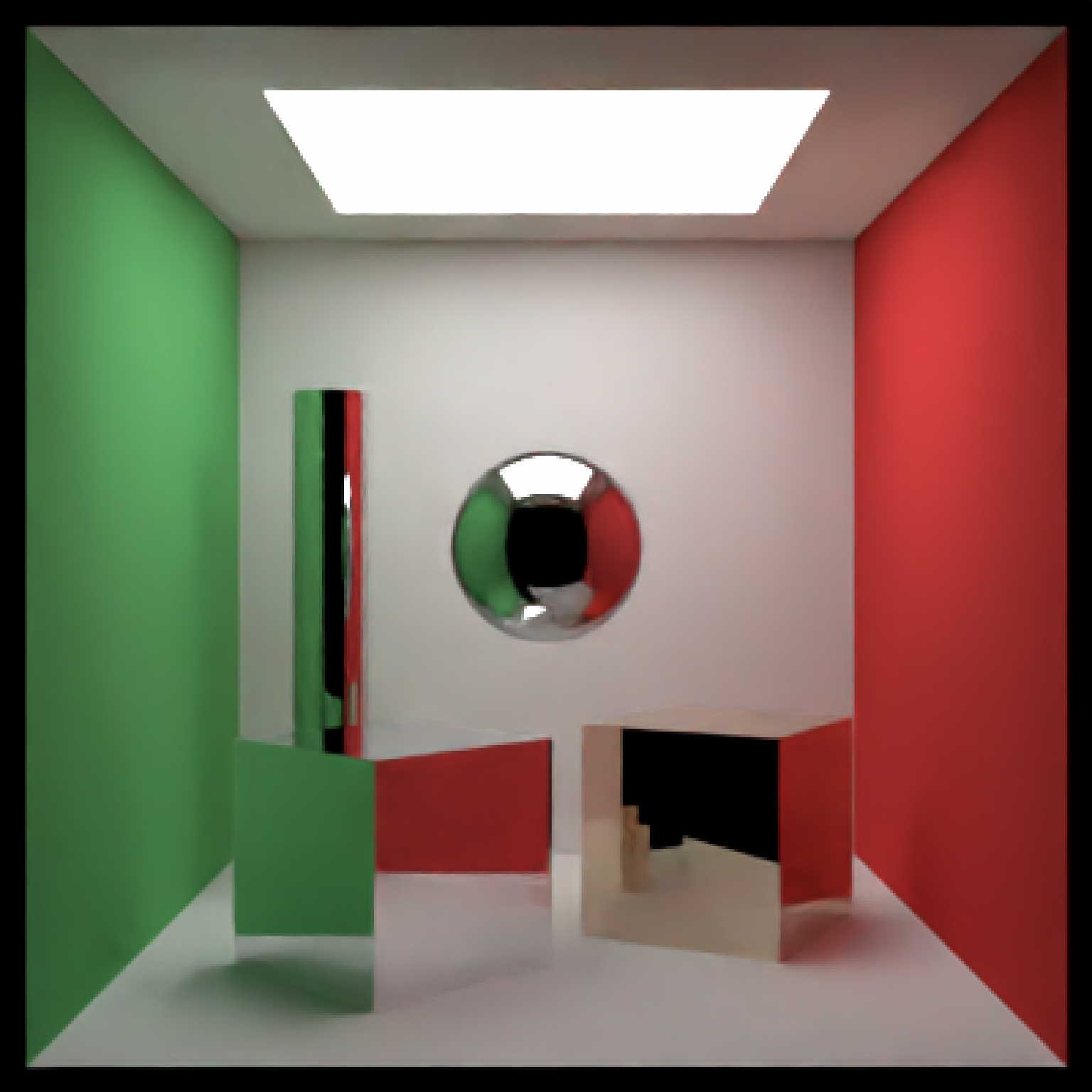Metallic Material

metal(
color = "#ffffff",
eta = 0,
kappa = 0,
fuzz = 0,
checkercolor = NA,
checkerperiod = 3,
noise = 0,
noisephase = 0,
noiseintensity = 10,
noisecolor = "#000000",
image_texture = NA,
image_repeat = 1,
alpha_texture = NA,
bump_texture = NA,
bump_intensity = 1,
importance_sample = FALSE
)

## Arguments

color Default white. The color of the sphere. Can be either a hexadecimal code, R color string, or a numeric rgb vector listing three intensities between 0 and 1. Default 0. Wavelength dependent refractivity of the material (red, green, and blue channels). If single number, will be repeated across all three channels. Default 0. Wavelength dependent absorption of the material (red, green, and blue channels). If single number, will be repeated across all three channels. Default 0. Deprecated--Use the microfacet material instead, as it is designed for rough metals. The roughness of the metallic surface. Maximum 1. Default NA. If not NA, determines the secondary color of the checkered surface. Can be either a hexadecimal code, or a numeric rgb vector listing three intensities between 0 and 1. Default 3. The period of the checker pattern. Increasing this value makes the checker pattern bigger, and decreasing it makes it smaller Default 0. If not 0, covers the surface in a turbulent marble pattern. This value will determine the amount of turbulence in the texture. Default 0. The phase of the noise. The noise will repeat at 360. Default 10. Intensity of the noise. Default #000000. The secondary color of the noise pattern. Can be either a hexadecimal code, or a numeric rgb vector listing three intensities between 0 and 1. Default NA. If not NA, creates a secondary color for a linear gradient between the this color and color specified in color. Direction is determined by gradient_transpose. Default FALSE. If TRUE, this will use the v coordinate texture instead of the u coordinate texture to map the gradient. Default NA. If not NA, this changes the behavior from mapping texture coordinates to mapping to world space coordinates. This should be a length-3 vector specifying the x,y, and z points where the gradient begins with value color. Default NA. If not NA, this changes the behavior from mapping texture coordinates to mapping to world space coordinates. This should be a length-3 vector specifying the x,y, and z points where the gradient begins with value gradient_color. Default hsv. Colorspace to calculate the gradient. Alternative rgb. Default NA. A 3-layer RGB array or filename to be used as the texture on the surface of the object. Default 1. Number of times to repeat the image across the surface. u and v repeat amount can be set independently if user passes in a length-2 vector. Default NA. A matrix or filename (specifying a greyscale image) to be used to specify the transparency. Default NA. A matrix, array, or filename (specifying a greyscale image) to be used to specify a bump map for the surface. Default 1. Intensity of the bump map. High values may lead to unphysical results. Default FALSE. If TRUE, the object will be sampled explicitly during the rendering process. If the object is particularly important in contributing to the light paths in the image (e.g. light sources, refracting glass ball with caustics, metal objects concentrating light), this will help with the convergence of the image.

## Value

Single row of a tibble describing the metallic material.

## Examples

# Generate the cornell box with a single chrome sphere in the center. For other metals,
# See the website refractiveindex.info for eta and k data, use wavelengths 5
# 80nm (R), 530nm (G), and 430nm (B).
scene = generate_cornell() %>%
material=metal(eta=c(3.2176,3.1029,2.1839), k = c(3.3018,3.33,3.0339))))
# \donttest{
render_scene(scene, lookfrom=c(278,278,-800),lookat = c(278,278,0), samples=50,
aperture=0, fov=40, ambient_light=FALSE, parallel=TRUE)# }
#Add an aluminum rotated shiny metal block
scene = scene %>%
material = metal(eta = c(1.07,0.8946,0.523), k = c(6.7144,6.188,4.95)),angle=c(0,45,0)))
# \donttest{
render_scene(scene, lookfrom=c(278,278,-800),lookat = c(278,278,0), samples=500,
aperture=0, fov=40, ambient_light=FALSE, parallel=TRUE)# }
scene = scene %>%
material = metal(eta = c(0.497,0.8231,1.338),
k = c(2.898,2.476,2.298)),
angle=c(0,-30,0)))
# \donttest{
render_scene(scene, lookfrom=c(278,278,-800),lookat = c(278,278,0), samples=500,
aperture=0, fov=40, ambient_light=FALSE, parallel=TRUE)# }# }